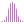#Percentile (PQL)

Returns the amount represented by the designated percentile amongst elements in the specified list.

#### Syntax

Percentile( <List> , <Integer> , OPTIONAL )

* Click on the function's arguments above for more details on the input values.

• List parameter should be a list or set of elements from an existing hierarchy in the model.
• The percentile value is a whole number from 0 to 100. For instance, '75' represents the 75th percentile (not '0.75').
• The data point or tuple is optional, and describes the values used to generate the percentile. If not supplied, the default measure or the query context will determine this value instead.
• For details on how to employ and use this function see the semantic calculation overview.
##### Different Function types
• This function is like the MDX median function, which calculates the 50th percentile across a list of elements in a hierarchy or attribute. However, this PQL function it provides more flexibility, enabling users to choose any percentile. Using 0 would effectively return the minimum value, while 100 would return the maximum value.
• Median, first and third quartile functions are available if you wish to change the aggregate of a numeric column in the database.
• To use a simple percentile mathematical operation on a list of numbers, use the Common library function.

#### Examples

This example returns the 50th Percentile expenses across the product category in the product table/ dimension is calculated, using the sample demo model:

Percentile( {AllMembers([products].[Product Category])}, 50, ([measures].[data Expenses]) )

Below, using the sample data of the 4 product categories we can use the above formula to calculate the 50th percentile (median). The list contains 4 values; the median, or middle value, will need to be resolved using interpolation between the 2 inner values (67,857 and 555,240), producing 311,549: